# Unit I: Electrostatics - Online Test

Q1. Two identical point charges of magnitude –q are fixed as shown in the figure below. A third charge +q is placed midway between the two charges at the point P. Suppose this charge +q is displaced a small distance from the point P in the directions indicated by the arrows, in which direction(s) will +q be stable with respect to the displacement?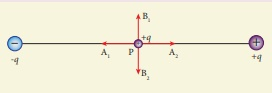Explaination / Solution:

Hint: Since V=0 along equatorial line
Q2. Which charge configuration produces a uniform electric field?
Explaination / Solution:
No Explaination.

Q3. What is the ratio of the charges |q1/q2| for the following electric field line pattern?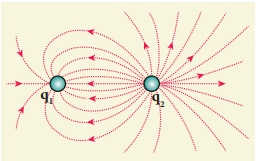Explaination / Solution:Q4. An electric dipole is placed at an alignment angle of 30º with an electric field of 2 × 105 N C-1. It experiences a torque equal to 8 N m. The charge on the dipole if the dipole length is 1 cm is
Explaination / Solution:Q5. Four Gaussian surfaces are given below with charges inside each Gaussian surface. Rank the electric flux through each Gaussian surface in increasing order.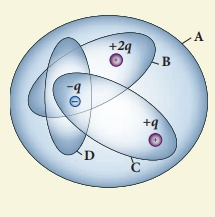Explaination / Solution:Q6. The total electric flux for the following closed surface which is kept inside water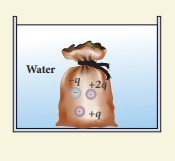Explaination / Solution: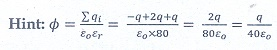Q7. Two identical conducting balls having positive charges q1 and q2 are separated by a center to center distance r. If they are made to touch each other and then separated to the same distance, the force between them will be
Explaination / Solution: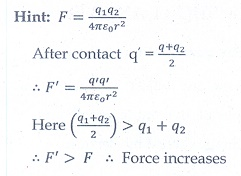Q8. Rank the electrostatic potential energies for the given system of charges in increasing order.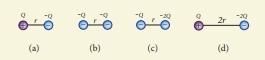Explaination / Solution: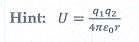Q9. An electric fieldexists in a certain region of space. Then the potential difference V = Vo – VA, where Vo is the potential at the origin and VA is the potential at x = 2 m is:
Explaination / Solution:Q10. A thin conducting spherical shell of radius R has a charge Q which is uniformly distributed on its surface. The correct plot for electrostatic potential due to this spherical shell is
Explaination / Solution:
No Explaination.